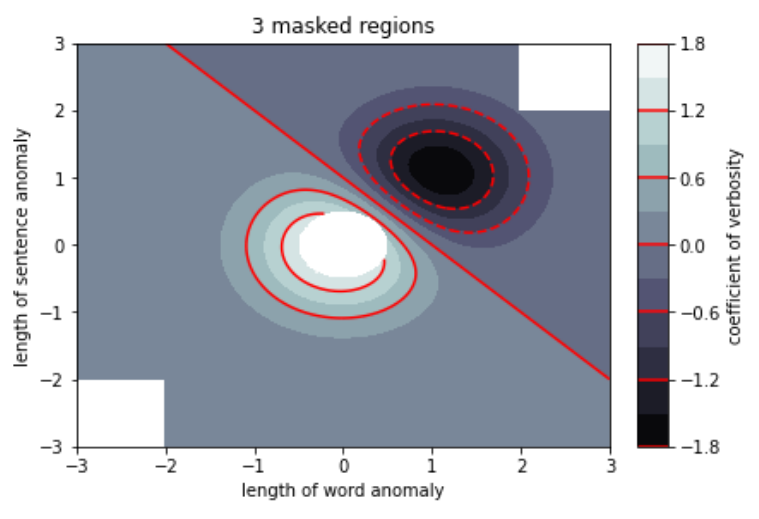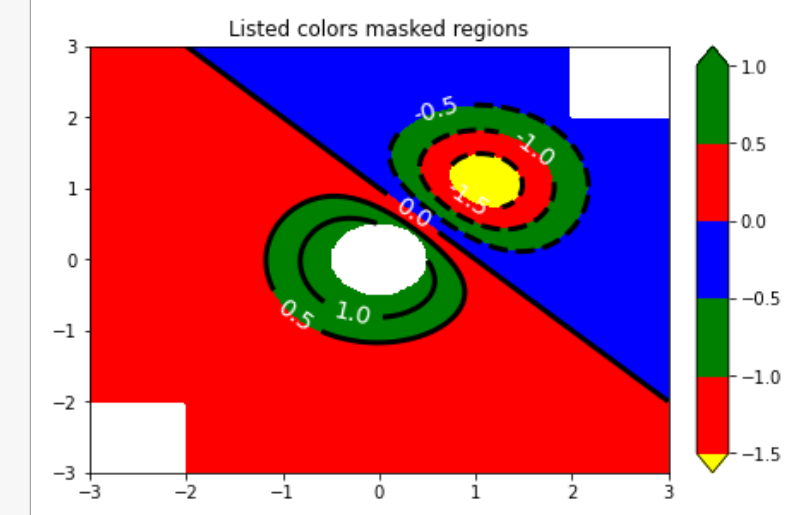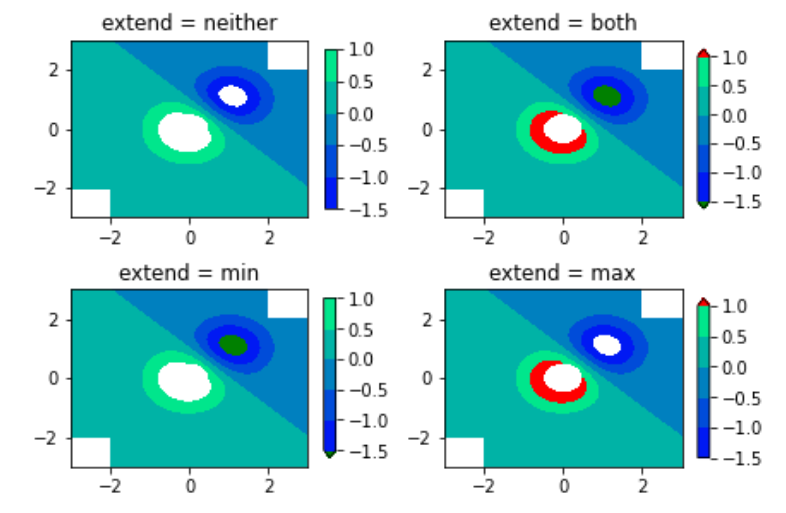# Matplotlib.colors.Colormap class in Python

Matplotlib is an amazing visualization library in Python for 2D plots of arrays. Matplotlib is a multi-platform data visualization library built on NumPy arrays and designed to work with the broader SciPy stack.

## matplotlib.colors.Colormap

The matplotlib.colors.Colormap class belongs to the matplotlib.colors module. The matplotlib.colors module is used for converting color or numbers arguments to RGBA or RGB.This module is used for mapping numbers to colors or color specification conversion in a 1-D array of colors also known as colormap.

The matplotlib.colors.Colormap class is a base class for all scalar to RGBA mappings. Generally colormap instance are used to transform data values (floats) from interval 0-1 to their respective RGBA color. Here the matplotlib.colors.Normalize class is used for scaling the data. The matplotlib.cm.ScalarMappable subclasses heavily use this for data->normalize->map-to-color processing chain.

Syntax:

class matplotlib.colors.Colormap(name, N=256)

Parameters:

• name: It accepts a string that represents the name of the color.
• N: It is an integer value that represents the number of rgb quantization levels.

Methods of Class:

1. colorbar_extend = None : If the colormap exists on a scalar mappable and colorbar_extend is set to false, colorbar_extend is picked up by colorbar creation as the default value for the extend keyword in the constructor of matplotlib.colorbar.Colorbar.
2. is_gray(self): Returns a boolean value to check if the plt is gray.
3. reversed(self, name=None): It is used to make a reversed instance of Colormap. This function is not implemented for the base class. It has a single parameter ie, name that is optional and and accepts a string name for the reversed colormap. If set to None the becomes the name of the parent colormap + “r”.
4. set_bad(self, color=’k’, alpha=None): It sets color that is to be used for masked values.
5. set_over(self, color=’k’,, alpha=None): It is used to set color to be used for high out-of-range values. It requires norm.clip to be False.
6. set_under(self, color=’k’,, alpha=None):It is used to set color to be used for low out-of-range values. It requires norm.clip to be False.

Example:

 `import` `numpy as np ` `import` `matplotlib.pyplot as plt ` ` `  `start_point ``=` `'lower'` ` `  `diff ``=` `0.025` ` `  `a ``=` `b ``=` `np.arange(``-``3.0``, ``3.01``, diff) ` `A, B ``=` `np.meshgrid(a, b) ` `X1 ``=` `np.exp(``-``A``*``*``2` `-` `B``*``*``2``) ` `X2 ``=` `np.exp(``-``(A ``-` `1``)``*``*``2` `-` `(B ``-` `1``)``*``*``2``) ` `X ``=` `(X1 ``-` `X2) ``*` `2` ` `  `RR, RC ``=` `X.shape ` ` `  `# putting NaNs in one corner: ` `X[``-``RR ``/``/` `6``:, ``-``RC ``/``/` `6``:] ``=` `np.nan ` ` `  `X ``=` `np.ma.array(X) ` `# masking the other corner: ` `X[:RR ``/``/` `6``, :RC ``/``/` `6``] ``=` `np.ma.masked ` ` `  `# masking a circle in the middle: ` `INNER ``=` `np.sqrt(A``*``*``2` `+` `B``*``*``2``) < ``0.5` `X[INNER] ``=` `np.ma.masked ` ` `  `# using automatic selection of ` `# contour levels; ` `figure1, axes2 ``=` `plt.subplots(constrained_layout ``=` `True``) ` `C ``=` `axes2.contourf(A, B, X, ``10``,  ` `                   ``cmap ``=` `plt.cm.bone,  ` `                   ``origin ``=` `start_point) ` ` `  `C2 ``=` `axes2.contour(C, levels ``=` `C.levels[::``2``],  ` `                   ``colors ``=``'r'``, origin ``=` `start_point) ` ` `  `axes2.set_title(``'3 masked regions'``) ` `axes2.set_xlabel(``'length of word anomaly'``) ` `axes2.set_ylabel(``'length of sentence anomaly'``) ` ` `  `# Make a colorbar for the ContourSet  ` `# returned by the contourf call. ` `cbar ``=` `figure1.colorbar(C) ` ` `  `cbar.ax.set_ylabel(``'coefficient of verbosity'``) ` ` `  `# Add the contour line levels ` `# to the colorbar ` `cbar.add_lines(C2) ` ` `  `figure2, axes2 ``=` `plt.subplots(constrained_layout ``=` `True``) ` ` `  `# making a contour plot with the ` `# levels specified, ` `levels ``=` `[``-``1.5``, ``-``1``, ``-``0.5``, ``0``, ``0.5``, ``1``] ` `C3 ``=` `axes2.contourf(A, B, X, levels, ` `                   ``colors ``=``(``'r'``, ``'g'``, ``'b'``), ` `                   ``origin ``=` `start_point, ` `                   ``extend ``=``'both'``) ` ` `  `# data below the lowest contour  ` `# level yellow, data below the ` `# highest level green: ` `C3.cmap.set_under(``'yellow'``) ` `C3.cmap.set_over(``'green'``) ` ` `  `C4 ``=` `axes2.contour(A, B, X, levels, ` `                  ``colors ``=``(``'k'``, ), ` `                  ``linewidths ``=``(``3``, ), ` `                  ``origin ``=` `start_point) ` ` `  `axes2.set_title(``'Listed colors (3 masked regions)'``) ` ` `  `axes2.clabel(C4, fmt ``=``'% 2.1f'``,  ` `             ``colors ``=``'w'``, ` `             ``fontsize ``=` `14``) ` ` `  `figure2.colorbar(C3) ` ` `  `# Illustrating all 4 possible  ` `# "extend" settings: ` `extends ``=` `[``"neither"``, ``"both"``, ``"min"``, ``"max"``] ` `cmap ``=` `plt.cm.get_cmap(``"winter"``) ` `cmap.set_under(``"green"``) ` `cmap.set_over(``"red"``) ` ` `  ` `  `figure, axes ``=` `plt.subplots(``2``, ``2``, ` `                            ``constrained_layout ``=` `True``) ` ` `  `for` `ax, extend ``in` `zip``(axes.ravel(), extends): ` `     `  `    ``cs ``=` `ax.contourf(A, B, X, levels,  ` `                     ``cmap ``=` `cmap,  ` `                     ``extend ``=` `extend,  ` `                     ``origin ``=` `start_point) ` `     `  `    ``figure.colorbar(cs, ax ``=` `ax, shrink ``=` `0.9``) ` `    ``ax.set_title(``"extend = % s"` `%` `extend) ` `    ``ax.locator_params(nbins ``=` `4``) ` ` `  `plt.show() `

Output:My Personal Notes arrow_drop_upCheck out this Author's contributed articles.

If you like GeeksforGeeks and would like to contribute, you can also write an article using contribute.geeksforgeeks.org or mail your article to contribute@geeksforgeeks.org. See your article appearing on the GeeksforGeeks main page and help other Geeks.

Please Improve this article if you find anything incorrect by clicking on the "Improve Article" button below.

Article Tags :

Be the First to upvote.

Please write to us at contribute@geeksforgeeks.org to report any issue with the above content.